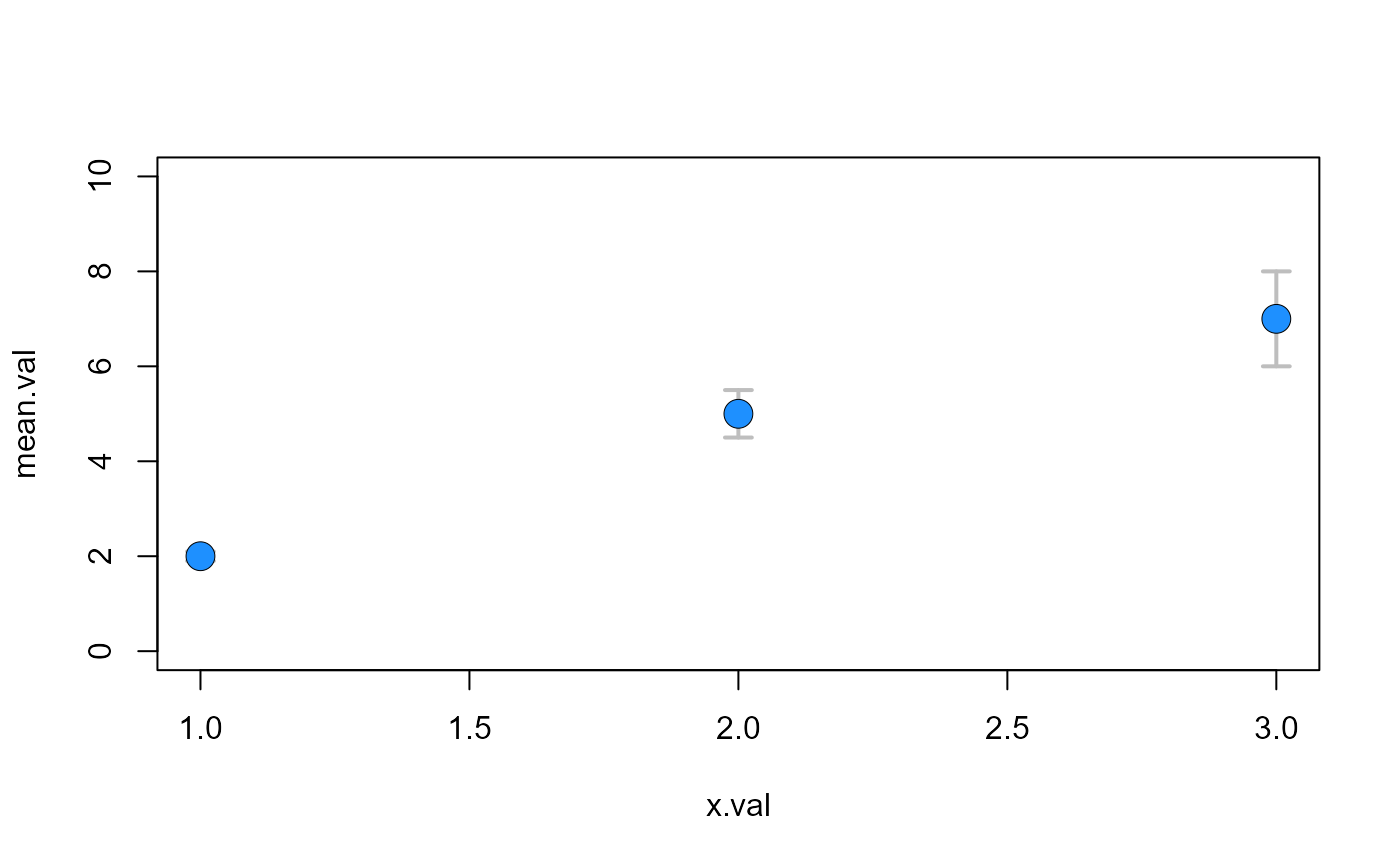Point with error bars

pt_error(
x,
y,
SE,
pch,
bg,
ln.col,
ln.lwd,
cex = 1,
pt.lwd = 0.5,
pt.lty = 1,
pt.col = "black",
length = 0.07
)

## Arguments

x x-value y-value (or mean) standard error plotting ‘character’, i.e., symbol to use. see points() background (fill) color for the open plot symbols given by pch = 21:25. see points() color of error bar lines width of error bar lines size of point line width of points line type for points color of point length of the edges of the error bar head (in inches).

## Value

draws lines in the form of error bars

## Note

see arrows() and errorbars() functions

## Examples

x.val=c(1,2,3)
mean.val=c(2,5,7)
se.val=c(0.1,0.5,1)
plot(mean.val~x.val,ylim=c(0,10),type="n")pt_error(x.val,mean.val,se.val,21,"dodgerblue1","grey",2,cex=2)